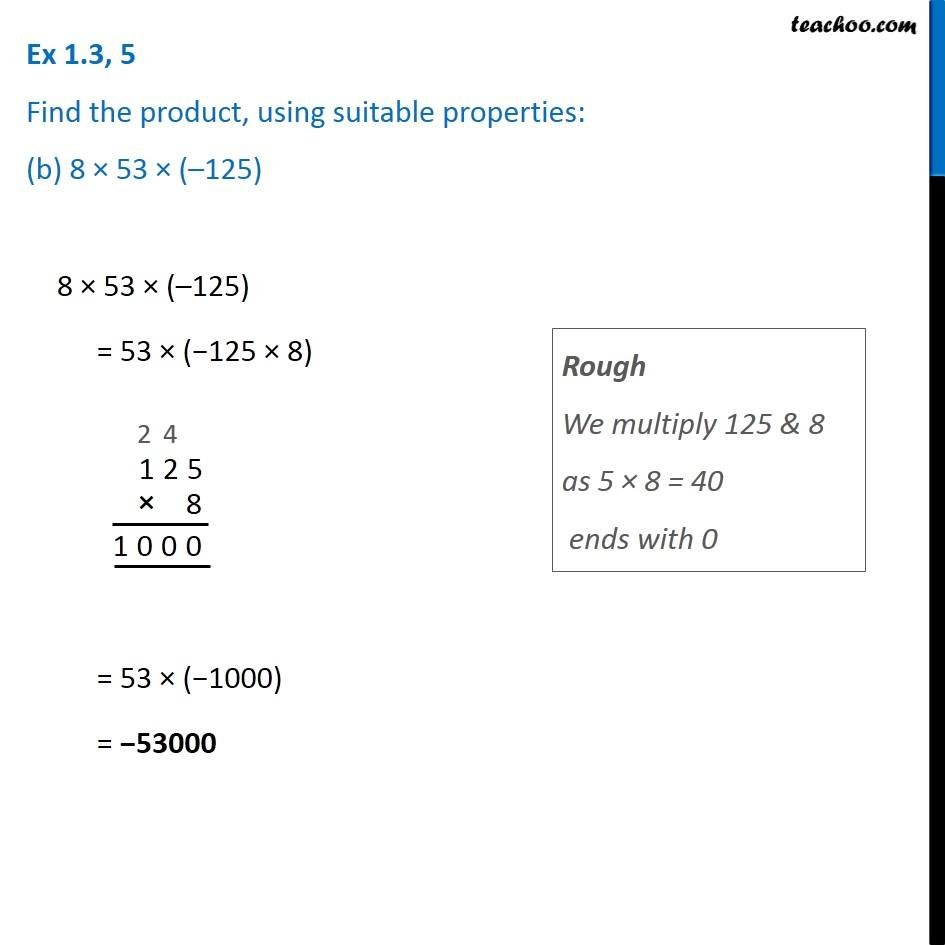Ex 1.2

Chapter 1 Class 7 Integers
Serial order wiseLearn in your speed, with individual attention - Teachoo Maths 1-on-1 Class

### Transcript

Ex 1.2, 5 Find the product, using suitable properties: (b) 8 × 53 × (–125) 8 × 53 × (–125) = 53 × (−125 × 8) Rough We multiply 125 & 8 as 5 × 8 = 40 ends with 0 = 53 × (−1000) = −53000